Binary Symplectic Form¶

Introduction¶

The qecc package provides support for elements of the Pauli and Clifford groups in binary symplectic form, including support for algorithms acting on these representations. Note that all classes and functions documented here depend on the numpy package. For more information on the binary symplectic representation, read [CRSS96], Section 2.

qecc.BinarySymplecticVector: Binary symplectic representation of Pauli group elements¶

The class qecc.BinarySymplecticVector provides a means of representing elements of the Pauli group (neglecting global phases) using binary vectors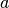and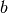such that an element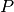of the Pauli group acting on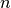qubits is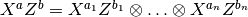. Binary symplectic vectors can be obtained from a single binary list, two binary lists, or converted from another Pauli instance (removing the phase):

>>> import qecc as q
>>> a=[1, 0, 1]; b=[0, 1, 1]
>>> q.BinarySymplecticVector(a,b)==q.BinarySymplecticVector(a+b)
True
>>> import qecc as q
>>> a=[1, 0, 1]; b=[0, 1, 1]
>>> q.BinarySymplecticVector(a,b)
( 1 0 1 | 0 1 1 )
>>> import qecc as q
>>> q.Pauli('XYIYIIZ',2).as_bsv()
( 1 1 0 1 0 0 0 | 0 1 0 1 0 0 1 )

Class Reference¶

class qecc.BinarySymplecticVector(*args)[source]

Encapsulates a binary symplectic vector representing an element of the Pauli group onqubits.

A new BinarySymplecticVector can be constructed using either a single NumPy array containing both the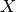and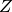parts of the binary symplectic vector. Alternatively, a new vector can be instantiated using two NumPy arrays. For example, the following two invocations are equivalent:

>>> import qecc
>>> import numpy as np
>>> bsv = qecc.BinarySymplecticVector(np.array([1, 0, 0, 0, 0, 0]))
>>> bsv = qecc.BinarySymplecticVector(np.array([1, 0, 0]), np.array([0, 0, 0]))

The len of a BinarySymplecticVector is defined as the number of qubits upon which the represented Pauli operator acts, and is thus half of the length of a single array containing the same data.

x

Array containing thepart of the binary symplectic vector.

Return type: numpy.ndarray, shape (2 * nq, ).
>>> import qecc as q
>>> q.BinarySymplecticVector([1,0,0,0,1,0]).x
array([1, 0, 0])
z

Array containing thepart of the binary symplectic vector.

Return type: numpy.ndarray, shape (nq, ).
>>> import qecc as q
>>> q.BinarySymplecticVector([1,0,0,0,1,0]).z
array([0, 1, 0])
copy()[source]

Returns a copy of the binary symplectic vector such that mutations of the copy do not affect this instance. For more details, see the numpy.ndarray.copy() method.

as_pauli()[source]

Returns an instance of qecc.Pauli representing the same Pauli operator as this vector. Note that phase information is not preserved by the binary symplectic representation of the Pauli group, and so P.as_bsv().as_pauli() need not equal P.

>>> import qecc as q
>>> pauli_with_phase=q.Pauli('IXXYZ',2)
>>> pauli_with_phase.as_bsv().as_pauli()
i^0 IXXYZ
bsip(other)[source]

Returns the binary symplectic inner product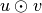of this vector with another vector. Letting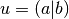and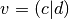,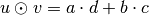.

>>> import qecc as q
>>> vector_a = q.BinarySymplecticVector([1,0,1],[0,1,1])
>>> vector_b = q.Pauli('YYZ').as_bsv()
>>> vector_a.bsip(vector_b)
1

Utility Functions¶

qecc.all_pauli_bsvs(nq)[source]

Lists all the Paulis on nq qubits according to their binary symplectic representations.

Parameters: nq (int) – Number of qubits. an iterator that yields the binary symplectic representations of each element of the Pauli group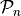.
>>> list(all_pauli_bsvs(1))
[( 0 | 0 ), ( 0 | 1 ), ( 1 | 0 ), ( 1 | 1 )]
qecc.constrained_set(pauli_array_input, logical_array_input)[source]

Given a set of constraints of the form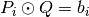, with each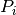a Pauli operator and each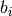a bit, yields an iterator onto Pauli operators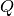such that all constraints are satisfied.

Parameters: pauli_array_input (list of qecc.Pauli instances.) – Constraint operators. logical_array_input (numpy.ndarray of dtype=int and shape (len(pauli_array_input), ).) – Constraint values.
>>> import qecc as q
>>> list(q.constrained_set(map(lambda s: q.Pauli(s).as_bsv(), ['XY','ZZ']),[1,0]))
[( 0 0 | 0 1 ), ( 0 0 | 1 0 ), ( 1 1 | 0 0 ), ( 1 1 | 1 1 )]
qecc.commute(bsv1, bsv2)[source]

Returns True if bsv1 and bsv2 commute by evaluating the symplectic inner product.

Return type: bool
qecc.xz_switch(bsv)[source]

Given a qecc.BinarySymplecticVector, returns a new vector whoseandparts have been swapped.

qecc.BinarySymplecticMatrix - Binary symplectic representation of Clifford group elements¶

Class Reference¶

class qecc.BinarySymplecticMatrix(*args)[source]

Encapsulates a binary symplectic matrix representing an element of the Clifford group onqubits.

A new BinarySymplecticMatrix can be constructed using either a single NumPy 2-D array containing the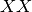,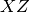,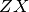, and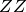parts of the binary symplectic matrix. Alternatively, a new matrix can be instantiated using four NumPy arrays. For example, the following two invocations are equivalent:

>>> import qecc
>>> import numpy as np
>>> bsm = qecc.BinarySymplecticMatrix(np.array([[1, 0, 0, 0],[1, 1, 0, 0],[0, 0, 1, 1],[0, 0, 0, 1]]))
>>> bsm = qecc.BinarySymplecticMatrix(np.array([[1, 0],[1, 1]]), np.array([[0, 0],[0, 0]]), np.array([[0, 0],[0, 0]]), np.array([[1, 1],[0, 1]]))
nq

Returns the number of qubits that the binary symplectic matrix acts upon.

xc

Returns the left half of a binary symplectic matrix.

zc

Returns the right half of a binary symplectic matrix.

xr

Returns the top half of a binary symplectic matrix.

zr

Returns the bottom half of a binary symplectic matrix.

xx

Returns the upper-left quadrant of a binary symplectic matrix.

xz

Returns the upper-right quadrant of a binary symplectic matrix.

zx

Returns the lower-left quadrant of a binary symplectic matrix.

zz

Returns the lower-right quadrant of a binary symplectic matrix.

left_H(j)[source]

Multiplies on the left by a Hadamard gate on the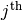qubit. This method acts in-place, as opposed to acting on a copy of the binary symplectic matrix. In order to preserve the original matrix, use the copy() method:

>>> new_bsm = bsm.copy().left_H(idx)
right_H(j)[source]

Multiplies on the right by a Hadamard gate on thequbit. See left_H() for more details.

right_H_all()[source]

Multiplies on the right by a Hadamard gate on each qubit. See left_H() for more details.

left_SWAP(j, k)[source]

Multiplies on the left by a SWAP gate between theand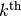qubits. This method acts in-place, as opposed to acting on a copy of the binary symplectic matrix. In order to preserve the original matrix, use the copy() method:

>>> new_bsm = bsm.copy().left_SWAP(j, k)
right_SWAP(j, k)[source]

Multiplies on the right by a SWAP gate between theandqubits. See left_SWAP() for more details.

left_CNOT(c, t)[source]

Multiplies on the left by a CNOT gate controlled by the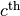qubit and targeting thequbit. This method acts in-place, as opposed to acting on a copy of the binary symplectic matrix. In order to preserve the original matrix, use the copy() method:

>>> new_bsm = bsm.copy().left_CNOT(c, t)
right_CNOT(c, t)[source]

Multiplies on the right by a CNOT gate controlled by thequbit and targeting thequbit. For more details, see left_CNOT().

left_R_pi4(i)[source]

Multiplies on the left by an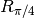gate acting on the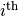qubit. This method acts in-place, as opposed to acting on a copy of the binary symplectic matrix. In order to preserve the original matrix, use the copy() method:

>>> new_bsm = bsm.copy().left_R_pi4(c, t)
right_R_pi4(i)[source]

Multiplies on the right by angate acting on thequbit. For more details, see left_R_pi4().

left_CZ(c1, c2)[source]

Multiplies on the left by an controlled-gate acting between the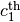and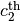qubits. This method acts in-place, as opposed to acting on a copy of the binary symplectic matrix. In order to preserve the original matrix, use the copy() method:

>>> new_bsm = bsm.copy().left_CZ(c, t)
right_CZ(c1, c2)[source]

Multiplies on the right by an controlled-gate acting between theandqubits. For more details, see left_CZ().

inv(check_validity=True)[source]

Returns the inverse of this binary symplectic matrix, assuming that this matrix represents a valid Clifford gate.

Note that if the matrix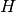does not represent a valid Clifford, this method will return a matrix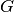such that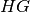is not the identity matrix.

Parameters: check_validity (bool) – If True, then the matrix is first checked to ensure that it is a valid Clifford. qecc.InvalidCliffordError if check_validity is True and the binary symplectic matrix being inverted does not represent a valid Clifford group element.
as_clifford(check_validity=True)[source]

Converts this binary symplectic matrix into a Clifford representation.

Parameters: check_validity (bool) – If True, then the matrix is first checked to ensure that it is a valid Clifford. qecc.Clifford The same gate as this binary symplectic matrix, represented as an instance of qecc.Clifford.
is_valid()[source]

Checks the satisfaction of the symplectic condition on a qecc.BinarySymplecticMatrix object.

copy()[source]

Returns a copy of this binary symplectic matrix, pointing to a distinct location in memory.

circuit_decomposition(validate=True)[source]

Decomposes the binary symplectic matrix using the algorithm of [AG04].

Utility Functions¶

qecc.is_bsm_valid(*args, **kwargs)[source]
qecc.bsmzeros(nq)[source]

Returns a binary symplectic matrix onqubits, initialized to all zeros.

Parameters: nq (int) – Number of qubits that the created matrix will act upon. A binary symplectic matrix containing all zeros. BinarySymplecticMatrix
qecc.array_to_pauli(bsv_array)[source]

Function wrapper for type conversion from binary symplectic vector to qecc.Pauli. See qecc.BinarySymplecticVector.as_pauli().